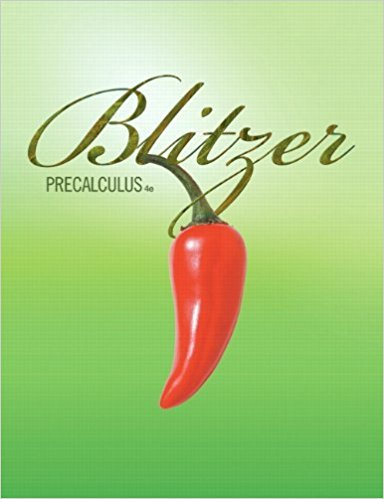×
×

# Solutions for Chapter Chapter 10: Sequences, Induction, and Probability## Full solutions for Precalculus | 4th Edition

ISBN: 9780321559845Solutions for Chapter Chapter 10: Sequences, Induction, and Probability

Solutions for Chapter Chapter 10
4 5 0 386 Reviews
12
1
##### ISBN: 9780321559845

Chapter Chapter 10: Sequences, Induction, and Probability includes 98 full step-by-step solutions. Since 98 problems in chapter Chapter 10: Sequences, Induction, and Probability have been answered, more than 70940 students have viewed full step-by-step solutions from this chapter. This expansive textbook survival guide covers the following chapters and their solutions. Precalculus was written by and is associated to the ISBN: 9780321559845. This textbook survival guide was created for the textbook: Precalculus, edition: 4.

Key Calculus Terms and definitions covered in this textbook
• Arccosecant function

See Inverse cosecant function.

• Average rate of change of ƒ over [a, b]

The number ƒ(b) - ƒ(a) b - a, provided a ? b.

• Categorical variable

In statistics, a nonnumerical variable such as gender or hair color. Numerical variables like zip codes, in which the numbers have no quantitative significance, are also considered to be categorical.

• Common logarithm

A logarithm with base 10.

• Compounded annually

See Compounded k times per year.

• Event

A subset of a sample space.

• Geometric series

A series whose terms form a geometric sequence.

• Head minus tail (HMT) rule

An arrow with initial point (x1, y1 ) and terminal point (x2, y2) represents the vector <8x 2 - x 1, y2 - y19>

• Horizontal line

y = b.

• Index of summation

See Summation notation.

• Intercept

Point where a curve crosses the x-, y-, or z-axis in a graph.

• Irrational numbers

Real numbers that are not rational, p. 2.

• Limaçon

A graph of a polar equation r = a b sin u or r = a b cos u with a > 0 b > 0

• Ordinary annuity

An annuity in which deposits are made at the same time interest is posted.

• Solve a triangle

To find one or more unknown sides or angles of a triangle

• Time plot

A line graph in which time is measured on the horizontal axis.

• Transformation

A function that maps real numbers to real numbers.

• Transitive property

If a = b and b = c , then a = c. Similar properties hold for the inequality symbols <, >, ?, ?.

• Whole numbers

The numbers 0, 1, 2, 3, ... .

• Ymin

The y-value of the bottom of the viewing window.

×